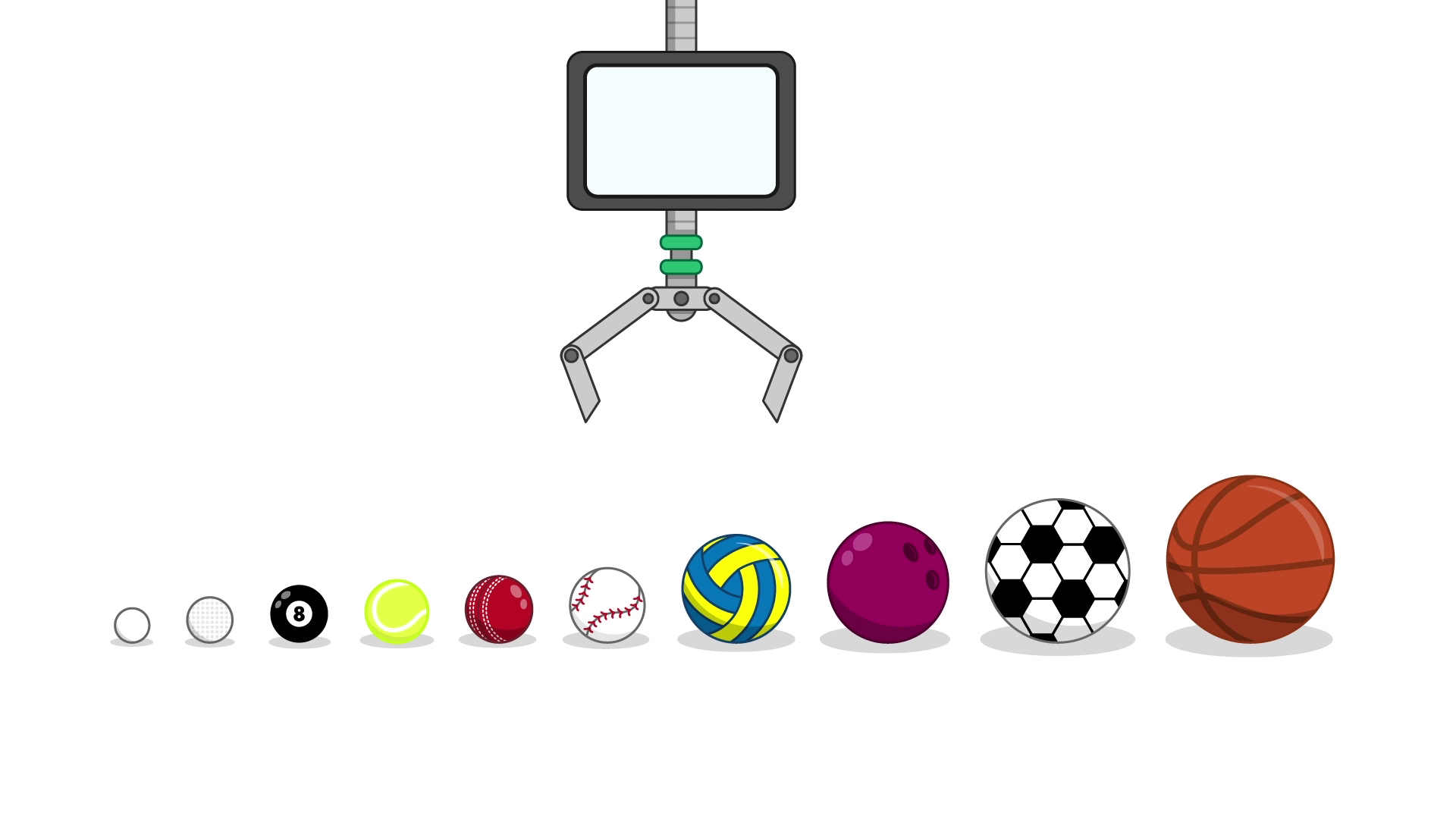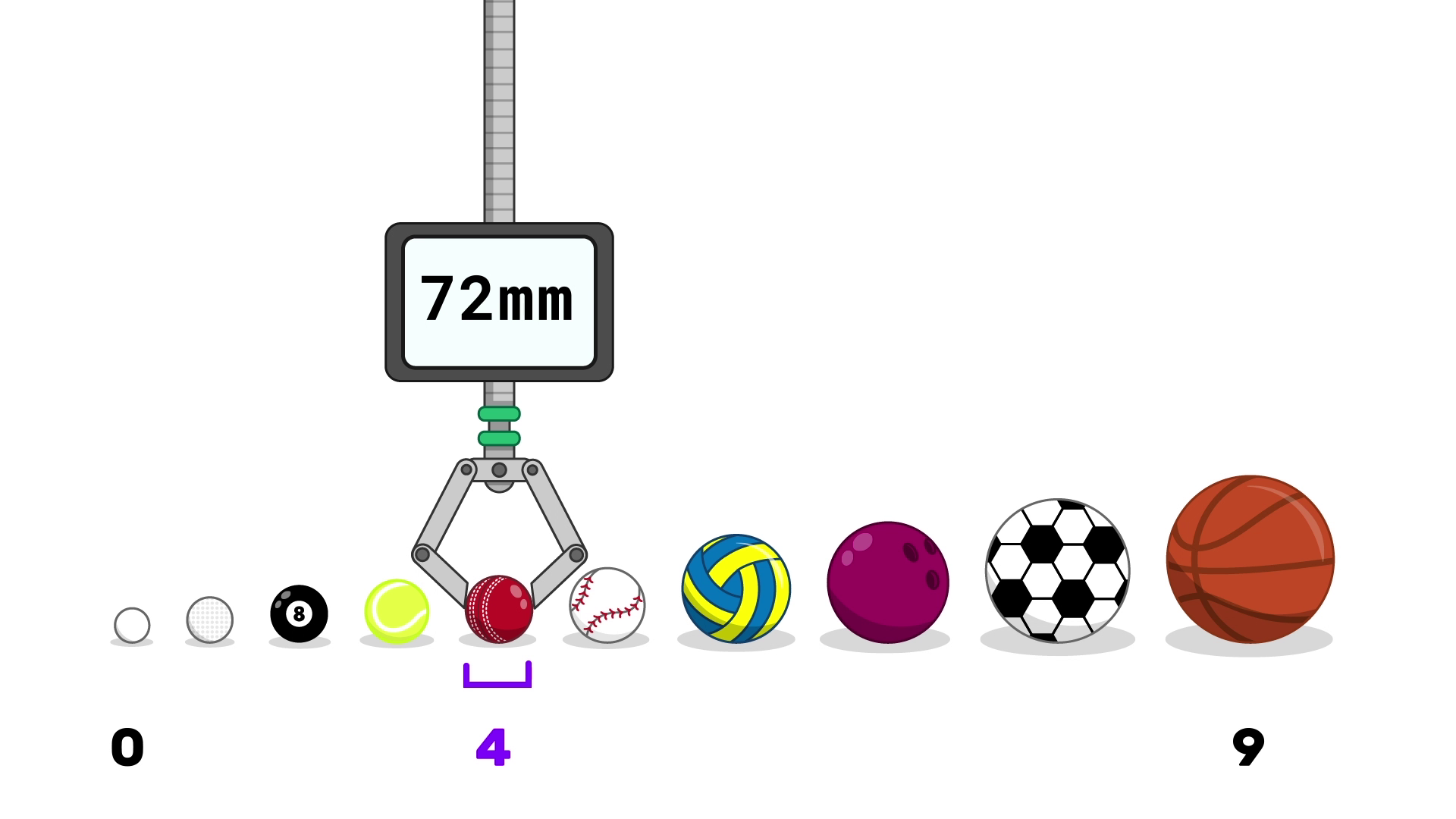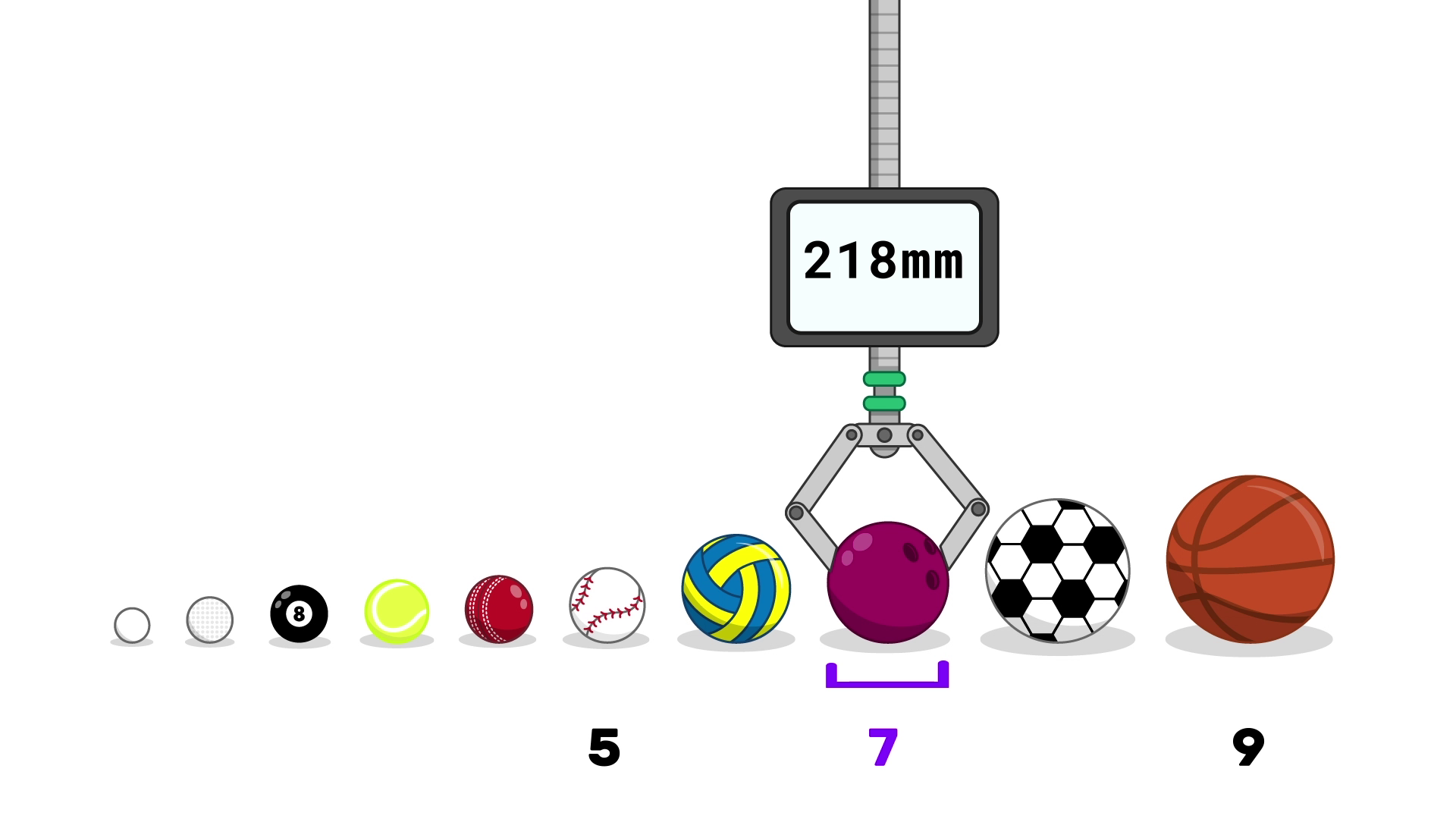## Want to keep learning?

This content is taken from the Raspberry Pi Foundation & National Centre for Computing Education's online course, Programming 102: Think Like a Computer Scientist. Join the course to learn more.
4.7

## Raspberry Pi Foundation

Skip to 0 minutes and 4 seconds Having looked at the fairly inefficient linear search algorithm, in this step, we’re going to look at a much more effective method for locating items in a sorted list.

Skip to 0 minutes and 14 seconds We can once again have a go at sorting out some sports balls. Imagine our programmed computer is in control of a robotic arm. We’ll line the balls up in a long shelf sorted from smallest to largest. Now imagine that the computer is told to fetch a bowling ball. The computer does a quick look up in a database, and sees that a bowling ball has a diameter of 218 millimetres. What would be your strategy for getting the robot arm to find the bowling ball in as few moves as possible? The binary search algorithm is perfect for this type of problem. After each iteration, the search becomes more and more focused.

Skip to 0 minutes and 55 seconds To do this, two positions are tracked– the lowest position to search from and the upper position to search to. As the computer hasn’t started the search yet, these values begin at 0 and 9. The computer is going to choose the first ball to search. To do this, it finds the midpoint of the two positions by adding them together and dividing by 2. In this case, the midpoint is 4.5, and so the computer selects the search position 4. It finds that the ball that has a diameter of 72 millimetres, making it a cricket ball. So the arm leaves the ball where it is. The computer determines that the bowling ball must lie further to the right.

Skip to 1 minute and 40 seconds That means that the new lower bound of the search becomes 5, and the upper position remains 9. Another calculation needs to be performed to find a new search position of the arm. Again, the midpoint of 5 and 9 is found, meaning our new search is at 7. The arm moves to position 7, which has a size of 218 millimetres. This matches the bowling ball, so the algorithm is complete, and the ball has been found. The bowling ball can be picked up and delivered to the user. Let’s have a look a search for a tennis ball, which is 67 millimetres. The arm moves to position 4 again, just like our first search.

Skip to 2 minutes and 22 seconds When it checks the ball there, it knows that a tennis ball is smaller, and so it must lie further to the left. The upper position now becomes 3. The midpoint of 0 and 3 is 1.5, so the search proceeds to position 1. The ball here is 43 millimetres, and so the tennis ball must be to the right. The lower bound now becomes 2. The midpoint of 2 and 3 as 2 and 1/2, so our new search position is 2. The ball here is measured to be 56 millimetres, hence the tennis ball must still be to the right. The lower bound becomes 3, meaning that both of our boundaries are 3, and therefore this must be our new search position.

Skip to 3 minutes and 5 seconds The ball is picked up, measured to be 67 millimetres, which is the tennis ball. It can now be picked up from the shelf. How efficient do you think this algorithm is? How many moves does it take if the ball that the computer is looking for isn’t in the collection of balls? How does the algorithm compare to the linear search, when the target ball is either the basketball or the table tennis ball? Share your thoughts in the comments section.

# Binary Search

We’ve seen how linear search can be made a bit more efficient if you know you are searching a sorted list. In this step, we’ll look at a much more efficient method for locating items in a sorted list.

The algorithm is called binary search, or sometimes binary chop. To understand how it works, let’s have a look at a fictitious example.

Once again, we’ll have a go at finding a specific sports ball. Imagine our programmed computer is in control of a robotic arm. The computer can’t visually recognise different sports balls, but the arm can measure the diameter of a ball.

The balls are lined up on a long shelf, sorted from smallest to largest.

0. table tennis - 40
1. golf - 43
2. pool - 56
3. tennis - 67
4. cricket - 72
5. baseball - 76
6. volleyball - 210
7. bowling - 218
8. soccer - 223Now imagine that the computer is told to fetch a bowling ball, which it knows has a diameter of 218mm.

What’s your strategy for getting the robot arm to find the bowling ball in as few tries as possible?

The binary search algorithm is perfect for this type of problem. It looks at a ball in the middle, and if that ball is too large (or too small), knows that it now only needs to look at balls further left (or right). We need to track two numbers: the leftmost position the computer still needs to search, and the rightmost position it still needs to search. As it hasn’t started searching yet, the left is initially zero and the right is nine (the number of balls).

To start, the computer needs to choose its first ball to check. To do this, it takes the average of the left and right values, by adding them and dividing by two: 0+9 is nine, and dividing by 2 gives four and a half. We can round in either direction. Let’s round downwards, so the first position to check will be position four.Sliding right to the ball at position four, the arm drops and measures the ball’s diameter. It finds that the ball there has a diameter of 72mm, making it a cricket ball, so the arm leaves the ball where it is.

Now the computer knows that the bowling ball has a diameter greater than 72mm, so it must lie further to the right. That means that the new leftmost position is five, and the rightmost position is still nine.

Another calculation needs to be performed to find a new search position of the arm. Again we take the average of left and right: half of (5+9) is seven, so the new search position is seven.The arm moves to position 7, drops, and measures the ball which has a diameter of 218mm. This matches the bowling ball, so the algorithm is complete and the ball has been found. The bowling ball can be sent to the user.

Now, let’s have a look at a search for the tennis ball, which is 67mm.

1. The left position is 0 and the right is 9. Averaging, (0 + 9) / 2 = 4.5.
2. The arm moves to position 4 and checks the ball, which is 72mm. This is too large so the tennis ball must be to the left.
3. The left position is still 0 and the right is now 3. Averaging, (0 + 3) / 2 = 1.5.
4. The arm moves to position 1 and checks the ball, which is 43mm. This is too small so the tennis ball must be to the right.
5. The left position is now 2 and the right is still 3. Averaging, (2 + 3) / 2 = 2.5.
6. The arm moves to position 2 and checks the ball, which is 56mm. This is too small so the tennis ball must be to the right.
7. The left right position are now both 3, so this is the new search position.
8. The arm moves to position 3, and checks this ball which is 67mm. The tennis ball has been found and can be returned.

How efficient do you think this algorithm is? How many moves does it take if the ball that the computer is looking for isn’t in the collection of balls? How does the algorithm compare to linear search when the target ball is the basketball or the table tennis ball? Share your thoughts in the comments.